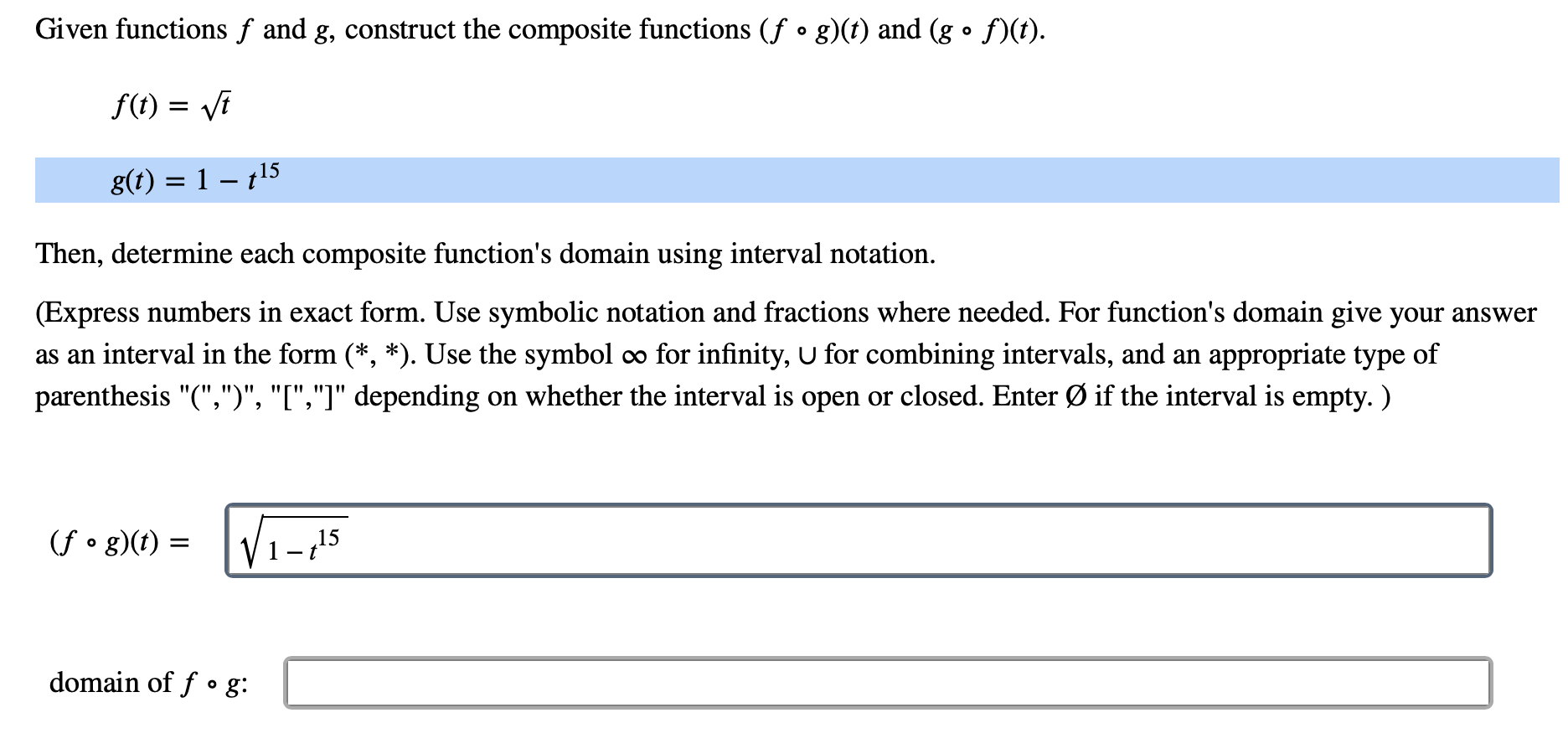# Given functions f and g, construct the composite functions (f ° g)(t) and (g ° f)(t)Of() ig(t) 1 -15Then, determine each composite function's domain using interval notation.(Express numbers in exact form. Use symbolic notation and fractions where needed. For function's domain give your answeras an interval in the form (*, *). Use the symbol0o for infinity, U for combining intervals, and an appropriate type ofon whether the interval is open or closed. Enter Ø if the interval is empty.)parenthesis "(",")", "I","]" depending(fo g)(f)15domain of fo g:

Question
1 viewshelp_outlineImage TranscriptioncloseGiven functions f and g, construct the composite functions (f ° g)(t) and (g ° f)(t) O f() i g(t) 1 -15 Then, determine each composite function's domain using interval notation. (Express numbers in exact form. Use symbolic notation and fractions where needed. For function's domain give your answer as an interval in the form (*, *). Use the symbol 0o for infinity, U for combining intervals, and an appropriate type of on whether the interval is open or closed. Enter Ø if the interval is empty.) parenthesis "(",")", "I","]" depending (fo g)(f) 15 domain of fo g: fullscreen
check_circle

Step 1

Given,

Step 2

Therefore,

Step 3

Now the domain of composite fu...

### Want to see the full answer?

See Solution

#### Want to see this answer and more?

Solutions are written by subject experts who are available 24/7. Questions are typically answered within 1 hour.*

See Solution
*Response times may vary by subject and question.
Tagged in

### Calculus